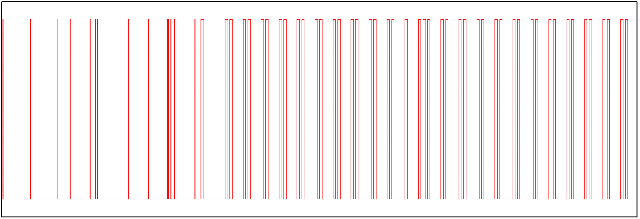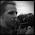## Description of the NEXA protocolOne burst of data from a NEXA remote.

The "new" NEXAprotocol are described here. Basically it is like this: The signal for start TX is a pulse (0.2 ms) followed by a delay of 2.7 ms. A pulse followed by a short delay (0.4 ms) reads as "0". A pulse followed by a longer delay (1.4 ms) reads as "1". The data are then combined into a 32 bit string by assigning "01" as '0' and "10" as '1'.

00000000001000000000000010010010

The sequence above were generated from a tellstick using "house=32770" and "unit=3".
The first 26 bits defines the ID of the remote as a binary number. If set, the 27th bit defines the command active for all recievers with the ID ( group command). The 28th bit are the command, '1' for "ON", '0' for "OFF". The last four bits are the unit code. For my NEXA remote control "unit 1" are "0000", "unit 2" are "0001" and "unit 3" are "0010". Every push on the remote generates a train of four pulse bursts, the tellstick generated five.

## DecodingIt is noisy in the 433 MHz range and hard to get the first burst of data.
It appears that the 433 MHz reciever used are doing level adjustment based on the signal level in the 433 MHz range so the strategy for initiating the decoding were to wait until some data forced the reciever to turn down the gain.

An Atmega 328P available at hand were chosen to do the decoding. The 433 MHz RX data pin were connected to one of the PCINT22 interrupt pins, PD6. The 16 bit timer1 were used to time pulse widths and delays. Power to the 433 MHz RX unit were provided from PD5 (GND) and PD7 (5V). PC5 were used for debugging by outputting pulses to a logic analyzer. Data were outputted to a LCD screen using code from extremeelectronics.

The 328P were run at 8 MHz. A check for how slow it can run and still decode the data are left for later.

`````` /*
*
* Sample signals from 433 MHz reciver and decode in a state machine.
*
*    PCB: SousVide V.1
*    Using Using port 11, 12 and 13 (PD5, PD6 and PD7) for 433 MHz RX, drawing ~4 mA
*
*
*
*
*
*/
#define F_CPU 8000000UL
#include <avr/io.h>
#include <avr/interrupt.h>
#include "lcd.h"
#define T01 1
#define T10 0
// shortest and longest accepted pulsewidth
#define kMinPulseWidth 140
#define kMaxPulseWidth 300 //210
// Minimum time between two pulsetrains
#define kPulseTrainDelay 9700
// Minimum time between init pulse and data stream
#define kMinInitDelay 2200 //2600
#define kMaxInitDelay 2800
// Maximum time between two data pulses
// A delay longer than reads as a '1'
#define kZeroDelay 687 //346
// The data arrives in pairs, '01' or '10', ministate
// keeps track of these pairs
//    -1    undefined, waiting for '0' or '1'
//     0    '0' read, waiting for '1'
//     1    '1'    read, waiting for '0'
volatile int8_t ministate = -1;
//#state
//# 0 - wait for 9.3 ms (kPulseTrainDelay) silence
//# 1 - wait for and measure init pulse width
//# 2 - measure init delay
//# 3 - check data pulse width and start measuring data delay
//# 4 - read data delay and determine '0', '1'. n time out store data
volatile uint8_t state = 0;
volatile uint32_t rawdata, data;
//PD5, ground for 433 MHz RX
#define RX_GND_PIN PD5
#define RX_DTA_PIN PD6
#define RX_VCC_PIN PD7
int main(void)
{
// Set up interrupts
PCMSK2 |= (1<<PCINT22);
PCICR |= (1<<PCIE2);
// Set up timer, do not start it
TCCR1B |= (1 << WGM12); //CTC mode
TIMSK1 |= (1<<OCIE1A);
state = 0;
rawdata = 0;
data = 0;
// Set up 433MHz RX
DDRD |= (1 << RX_GND_PIN) | (1 << RX_VCC_PIN);
DDRD &= ~(1<<RX_DTA_PIN);
PORTD &= ~(1 << RX_GND_PIN); // set to 0V
PORTD |= (1 << RX_VCC_PIN);    // set to 5V
//    PORTD |= (1 << RX_DTA_PIN); // pullup
// For debugging
DDRC |= (1 << PC5);
//Initialize LCD module
LCDClear();
sei();
while(1)
{
{
cli();
char buff;
uint8_t tmp = data &0x000000FF;
sprintf(buff,"%04X", (data>>(16+6))&0x0000FFFF);
LCDWriteStringXY(0,0,buff);
sprintf(buff,"%04X", (data>>6)&0xFFFF);
LCDWriteStringXY(4,0,buff);
if (tmp & 0x10)
{
LCDWriteStringXY(0,1,"ON");
}
else
{
LCDWriteStringXY(0,1,"OFF");
}
//        LCDWriteStringXY(4,1,"U ");
//        LCDWriteIntXY(6,1,(tmp&0x0f),3);
if ((tmp & 0x0F) == 0x00) LCDWriteStringXY(4,1,"U 1");
if ((tmp & 0x0F) == 0x01) LCDWriteStringXY(4,1,"U 2");
if ((tmp & 0x0F) == 0x02) LCDWriteStringXY(4,1,"U 3");
if (tmp & 0x20) LCDWriteStringXY(4,1,"Grp");
sei();
for (int ii=0;ii<100;ii++)
{
_delay_ms(30);
}
cli();
LCDClear();
sei();
}
}
}
//reset state to initial
void inline resetstate()
{
unsigned char sreg;
state=0;
ministate=-1;
data = 0;
rawdata = 0;
TCCR1B &= ~(1 << CS11); //stop timer 1
sreg = SREG; // save global interrupt flag
cli();
TCNT1 = 0;
SREG = sreg; // restore interrrupt flag
}
// start timer
void inline starttimer(int v)
{
unsigned char sreg;
sreg = SREG; // save global interrupt flag
cli();
TCNT1 = 0;
OCR1A = v;
SREG = sreg; // restore interrrupt flag
TCCR1B |= (1 << CS11); //prescale 8
}
// This interrupt is called when the 433 MHz RX pin changes state
ISR(PCINT2_vect)
{
char transition;
// determine the state change direction, 0->1 or 1->0
if (PIND & (1<<PIND6))transition = T01;
else transition = T10;
//state 0: should time out, we need 9.3 ms silence, otherwise try again
if (state == 0)
{
starttimer(kPulseTrainDelay-100);
return;
}
// state 1: we had a 9.3 ms delay, now we are recieving the init pulse
// start the pulsewidth timer
if ((state==1) && (transition == T01))
{
starttimer(kMaxPulseWidth);
return;
}
if ((state==1) && (transition == T10))
{
if (TCNT1<kMinPulseWidth)
{
resetstate();
return;
}
else // pulse OK, too long and it will time out in the timer part
{
state = 2;
starttimer(kMaxInitDelay);
return;
}
}
// state 2: measure the init delay
if (state == 2)// && (transition == T01))
{
if (transition == T10)
{
resetstate();
return;
}
if (TCNT1<kMinInitDelay) // to short
{
resetstate();
return;
}
else // delay OK, too long and it times out
{
starttimer(kMaxPulseWidth);
rawdata = 0;
state = 3;
return;
}
}
//state 3: check pulsewidth in data transmission
if ((state == 3))// && (transition==T10))
{
if (TCNT1<kMinPulseWidth)
{
resetstate();
return;
}
else // pulse OK, too long and it will time out from the timer
{
state = 4;
return;
}
}
//state 4: read data, '0' or '1'
if (state == 4)
{
int t = TCNT1;
//observing 1310 or 320
if (t > kZeroDelay) // we read '1'
{
if (ministate==1) // error, two '1' in a row
{
resetstate();
return();
}
else if (ministate==-1) // OK, next bit should be '0'
{
ministate = 1;
}
else if (ministate==0) // OK, bit pair completed
{
ministate = -1;
rawdata = (rawdata<<1);
// no need to add '0'
}
}
{
if (ministate==0) // error, two '0' in a row
{
resetstate();
return();
}
else if (ministate==-1) // OK, next bit should be '1'
{
ministate = 0;
}
else if (ministate==1) // OK, bit pair completed
{
ministate = -1;
rawdata = (rawdata<<1);
rawdata +=1;
}
}
state = 3;
starttimer(kMaxPulseWidth);
return;
}
}
ISR(TIMER1_COMPA_vect)
{
if (state==0)
{
state = 1;
TCCR1B &= ~(1 << CS11); //stop the timer
return;
}
// pulsewidth/delay were to long
if ((state == 1) || (state == 2) || (state == 3))
{
PORTC = 0b00100000;
PORTC = 0b00000000;
_delay_us(5);
PORTC = 0b00100000;
PORTC = 0b00000000;
resetstate();
return;
}
// end of data burst, wait for next burst
if (state==4)
{
data = rawdata;
rawdata = 0;
starttimer(kPulseTrainDelay>>1);
state = 0;
}
}
``````

This extracts the data in the pulsetrain, but it does not do any accumulation of the data in the pulse trains. Usaully there are at least four bursts that contains the same data.

#### 1 comment:

1.This worked great, I'm having some slight issues with reliability, but i think that comes from noise. (using crappy receiver from ebay).

Allthough PIN_RX is defined at the top, you are still using PIND6 somewhere in the code. Future users should stick to using PORTD pin 6 for RX.